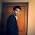# Check if a number is prime with Excel

Prime number
A prime number (or a prime) is a natural number greater than 1 that has no positive divisors other than 1 and itself.

Logic

Divide a number by each number between 2 and square root of the number. If the number has no factors less than its square root, then n is prime.

Example

Let's take a number 53 .

1. Calculate square root of 53 i.e. 7.28
2. Round 7.28 up i.e. 8
3. Divide 53 by each number between 2 and 8
(2,3,4,5,6,7,8)
4. You'll see there is always a remainder when 53 is divided by each number 2 and 8
5. Result : Prime

Excel Formula

Suppose a value is entered in cell B4 . Paste the below formula in cell C4.

Hit CTRL + SHIFT + ENTER to confirm this formula as an array formula. If done correctly, Excel will automatically place curly braces {...} around the formula.

Related Post

Counting Prime Numbers in an Interval with Excel
Generating Prime Numbers in an Interval with ExcelRelated PostsDeepanshu founded ListenData with a simple objective - Make analytics easy to understand and follow. He has over 8 years of experience in data science. During his tenure, he has worked with global clients in various domains like Banking, Insurance, Telecom and Human Resource.

16 Responses to "Check if a number is prime with Excel"
1.Not working for 9. Problem with some indirect addressing.

1.2.Your formula references A2, but doesn't say what I should be putting there. It's great that your formula works for your specific workbook, but if it can't be easily copied into another, then what is the point of publishing it.

3.Anonymous, it is very easily copied into any workbook, and it works exactly as advertised. The A2 reference makes no difference - a little investigation would have shown you that. And on that note, even if the formula wasn't directly translatable, would it really hurt to have to -gasp!- do a little of your own homework? There's a lot to learn from this post - that would be "the point" of publishing it.

2.Doesn't seem to be 100% accurate, for example, For the input I entered 111,111,111. It said it was prime, although it can be divided by 9 and 3 at least. Any idea what may cause this kind of behavior? I don't really understand how the function works, so I can't diagnose the problem myself.

1.It says 'Not Prime' for 111,111,111. You can save your workbook to any cloud drive and share link with me to debug.

3.This comment has been removed by the author.

1.This comment has been removed by the author.

2.This comment has been removed by the author.

3.This comment has been removed by the author.

4.Clever!! I never would have thought of using an array formula iterating along a virtual array to create a "for loop" inside an excel formula! I'm definitely going to have to remember that technique in the future!
Though, as evidenced by the comments, that technique is extremely advanced (and thus confusing) for most people. Perhaps a link to a post with an explanation of the technique would help.

5.Careful using this with numbers 81 and larger...

1.It works fine for me. Make sure to press CTRL + SHIFT + ENTER after entering the formula but BEFORE clicking away. You will know that you entered it correctly if selecting the cell shows
{=IF(B4=2,"Prime",IF(AND(MOD(B4,ROW(OFFSET(\$A\$2,,,ROUNDUP(SQRT(B4),0)-1)))<>0),"Prime","Not Prime"))}
note the {} brackets.
Also note that entering the brackets manually does NOT work; you have to press CTRL + SHIFT + ENTER to get the brackets.

6.Shows 6 as a prime, not correct

7.Thank you that is genius, works fine for me, shows 6 and 9 as prime.

8.74 Prime
75 Not Prime
76 Not Prime
77 Not Prime
78 Not Prime
79 Prime
80 Not Prime
81 Not Prime
82 Prime
83 Prime
84 Not Prime
85 Not Prime
86 Prime
87 Not Prime
88 Not Prime
89 Prime
90 Not Prime
91 Not Prime
92 Not Prime
93 Not Prime
94 Prime

Next → ← Prev
Love this Post? Spread the Word!
Share